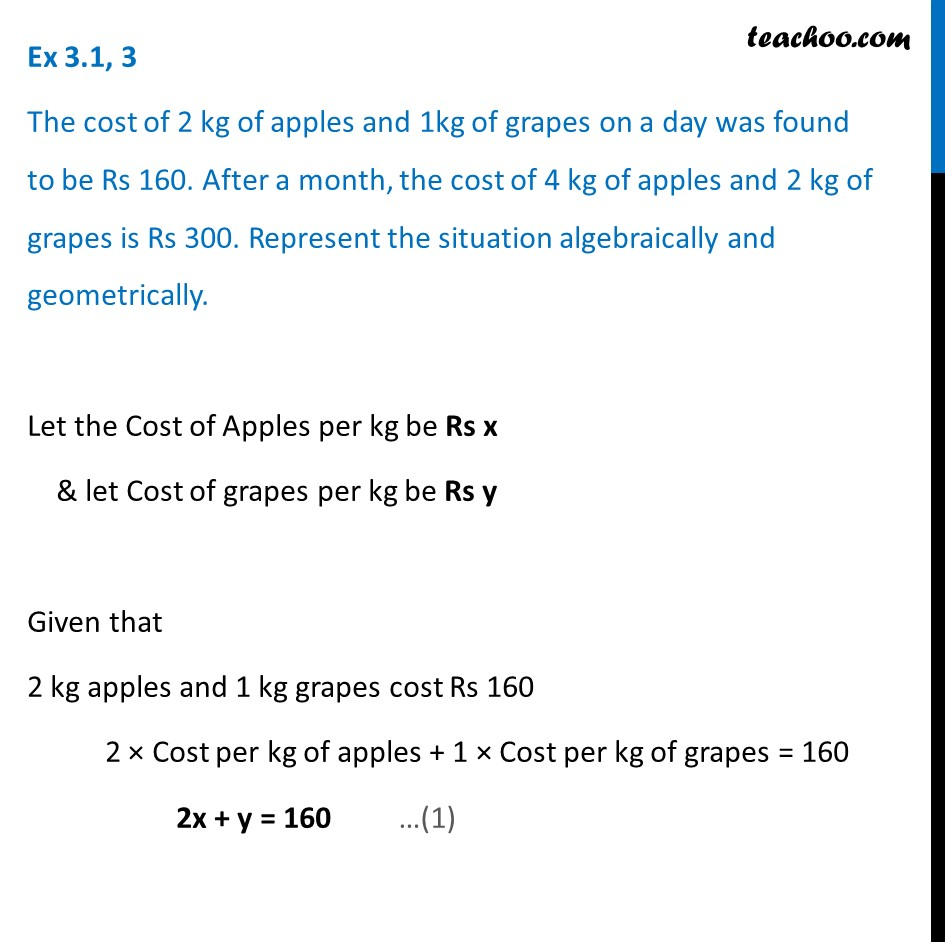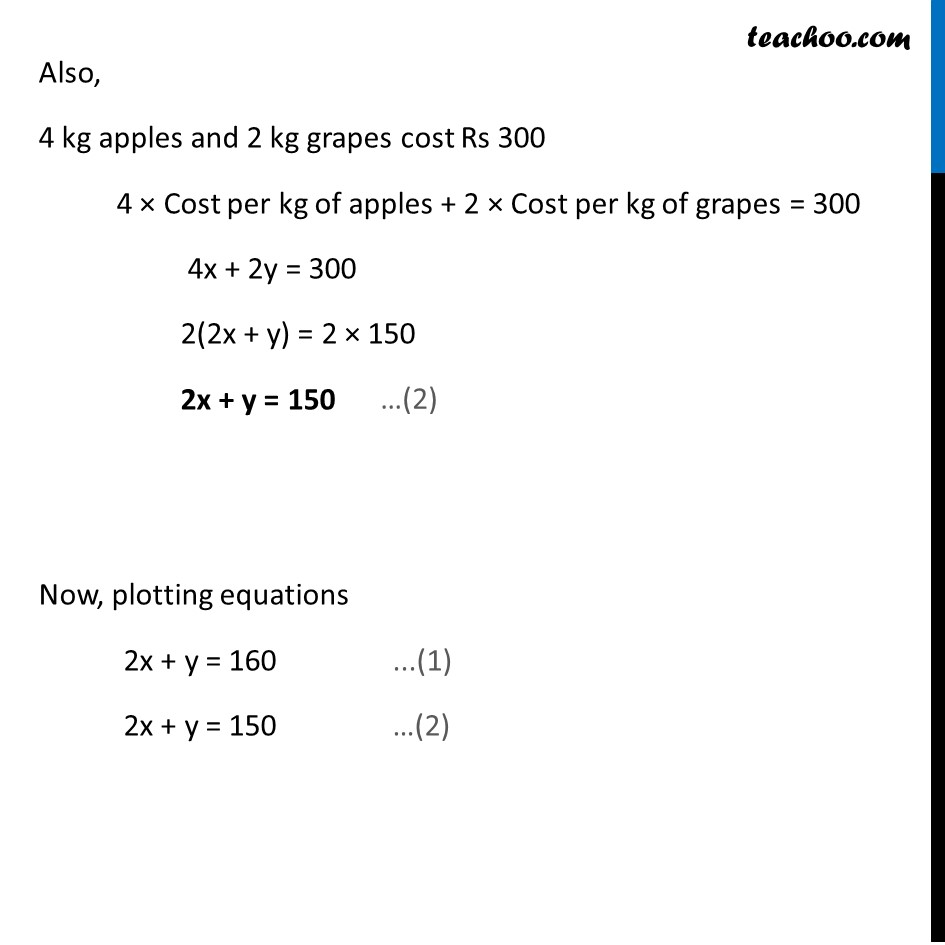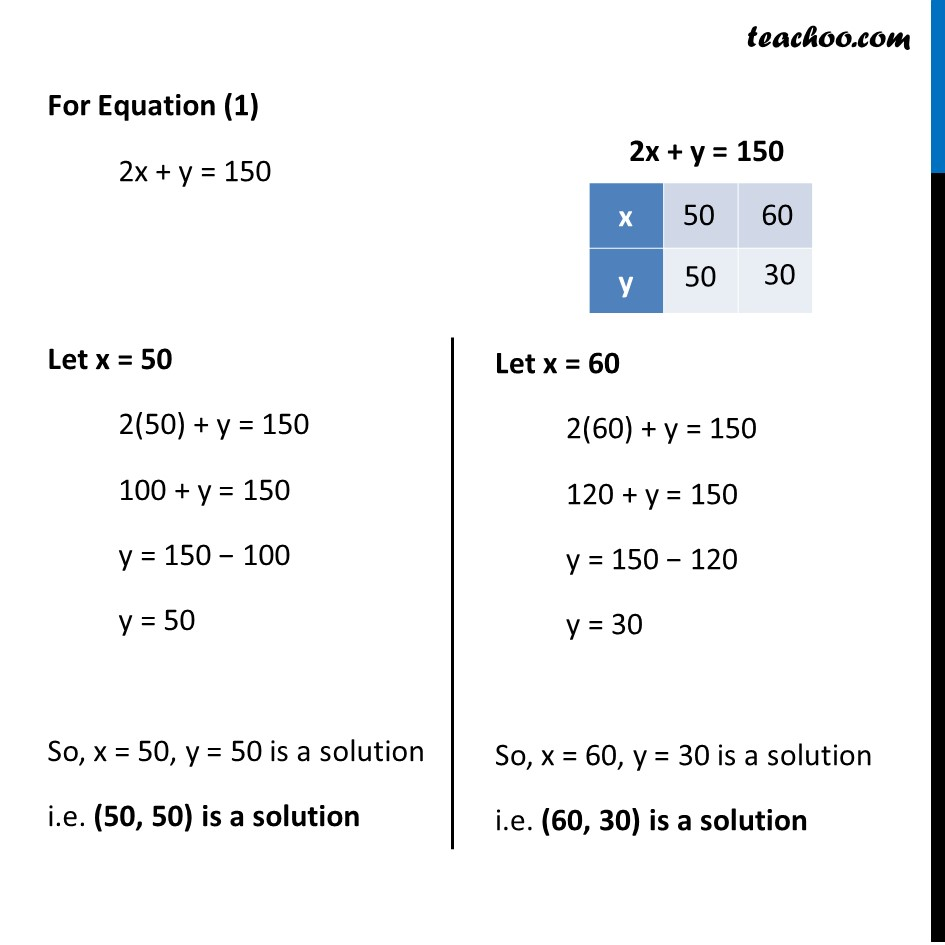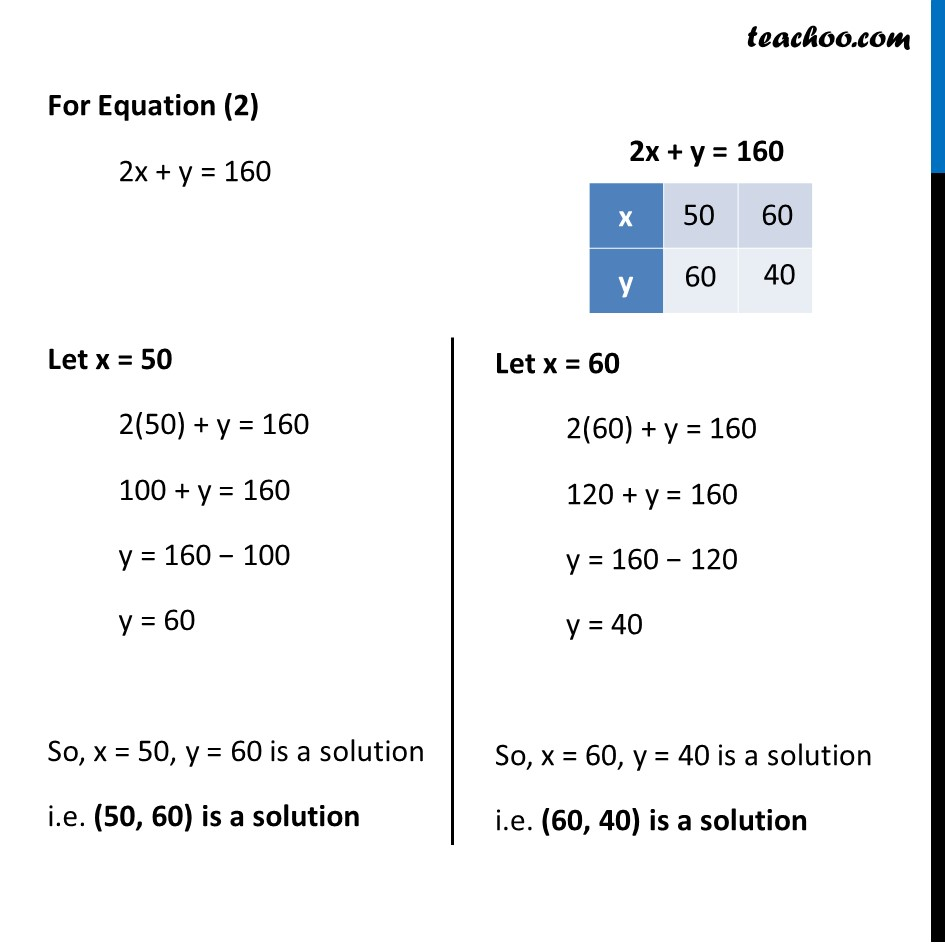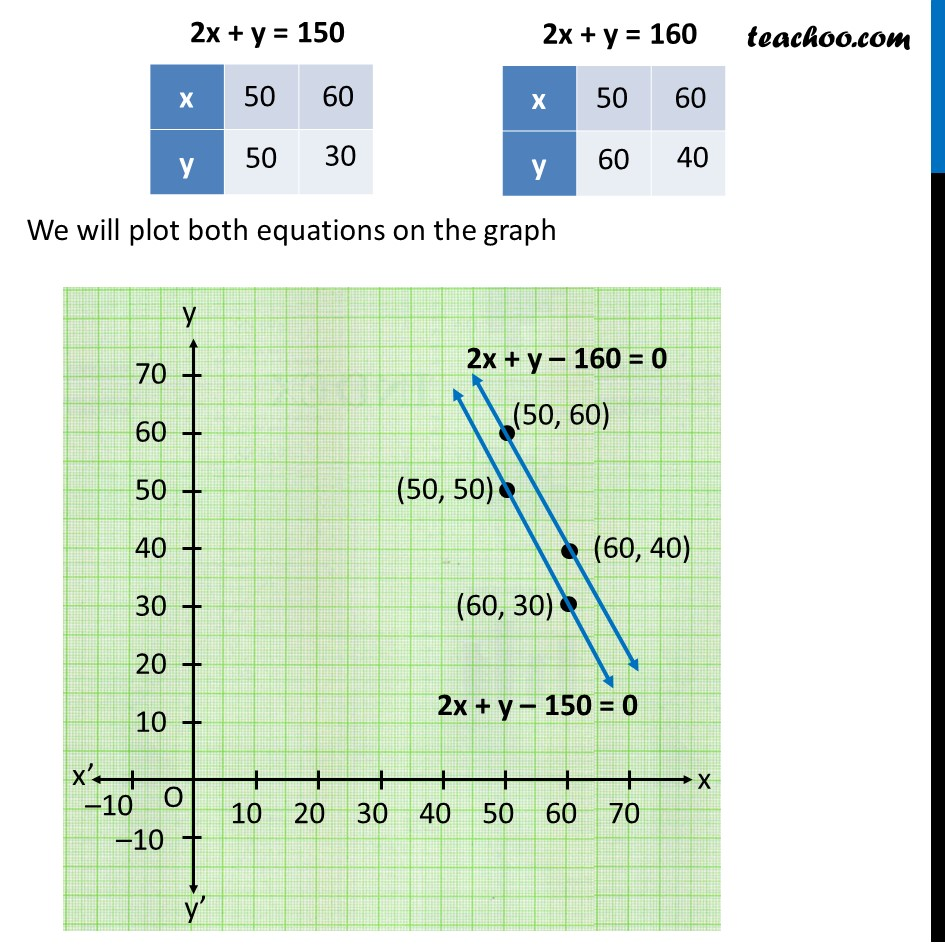1. Chapter 3 Class 10 Pair of Linear Equations in Two Variables (Term 1)
2. Concept wise
3. Forming equations graphically and algebraically

Transcript

Ex 3.1, 3 The cost of 2 kg of apples and 1kg of grapes on a day was found to be Rs 160. After a month, the cost of 4 kg of apples and 2 kg of grapes is Rs 300. Represent the situation algebraically and geometrically. Let the Cost of Apples per kg be Rs x & let Cost of grapes per kg be Rs y Given that 2 kg apples and 1 kg grapes cost Rs 160 2 × Cost per kg of apples + 1 × Cost per kg of grapes = 160 2x + y = 160 Also, 4 kg apples and 2 kg grapes cost Rs 300 4 × Cost per kg of apples + 2 × Cost per kg of grapes = 300 4x + 2y = 300 2(2x + y) = 2 × 150 2x + y = 150 Now, plotting equations 2x + y = 160 ...(1) 2x + y = 150 …(2) For Equation (1) 2x + y = 150 Let x = 50 2(50) + y = 150 100 + y = 150 y = 150 − 100 y = 50 So, x = 50, y = 50 is a solution i.e. (50, 50) is a solution Let x = 60 2(60) + y = 150 120 + y = 150 y = 150 − 120 y = 30 So, x = 60, y = 30 is a solution i.e. (60, 30) is a solution For Equation (2) 2x + y = 160 Let x = 50 2(50) + y = 160 100 + y = 160 y = 160 − 100 y = 60 So, x = 50, y = 60 is a solution i.e. (50, 60) is a solution Let x = 60 2(60) + y = 160 120 + y = 160 y = 160 − 120 y = 40 So, x = 60, y = 40 is a solution i.e. (60, 40) is a solution We will plot both equations on the graph